#### Series and Parallel Circuits

Type of Circuits
There are basically two types of circuits:
• Series Circuits
• Parallel Circuits
Series Circuits
In series circuits there is one path for current flow.
This is an example of series circuit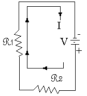Parallel circuits
In parallel circuits there could be two or more paths for current flow
This is an example of a parallel circuitWe use standard symbols to draw electronic circuits or schematics.
Here are some of the standard symbols used.
 Component Symbol Light Bulb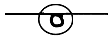Resistor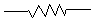Battery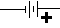Diode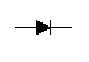LED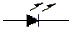SwitchesWire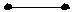REVIEW EXERCISES
A. Find the resistance for the following resistors and state the high/low range for each.
1. brown, black, red, gold
2. orange, yellow, red, silver
3. blue, black, green, silver
B.Draw a circuit diagram, using proper symbols to show the following
1. A series circuit with a battery, an ammeter, and a light bulb
2. A parallel circuit with a battery, a voltmeter, an a light bulb